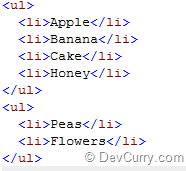# jQuery: Numbering an Unordered List

 Tweet
Here’s a very simple script which adds numbers sequentially to an unordered list using jQuery
Let us assume you have an unordered list as shown below:To add numbers to it sequentially, use this code:

```<script type="text/javascript"
src="http://ajax.aspnetcdn.com/ajax/jQuery/jquery-1.6.1.min.js">
</script>
<script type="text/javascript">
\$(function () {
\$('ul > li').each(function () {
\$(this).prepend("(" +
(\$(this).index() + 1) + ") ");
});
})
</script>
```

As you can see, we are using the jQuery Prepend function to insert numbers to the beginning of each element in the set of matched elements.

OUTPUT